# Round to Nearest 100 in Microsoft Excel 2010

While preparing the Income Statement reports such as ‘Net Sales’, you can see if the company’s sales are improving over time by comparing the current year’s figure to previous year’s figures.

For example, imagine looking at the Income Statement with the following amounts over the past two years: \$4,716,917&\$4,502,079. Now look at the amounts rounded to the nearest thousand: \$4,717&\$4,502. The rounded figures allow you to focus on the relevant digits. The rounded amounts also make it easier to see the trend.

To round a number we can use the Round, Roundup, Rounddown functions.We can round a number to a specific number of digits by using Custom Formats too.

Rounding Numbers to Specific Number using Custom Format

Column A contains few numbers which need to be rounded down to two digits.

To achieve this -

• Select cells in column A &right click on the mouse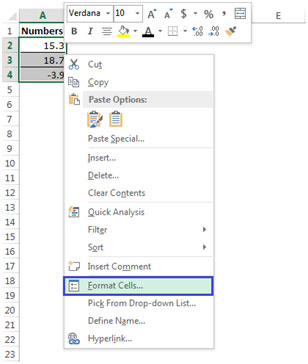• Click on Format Cells orPress Ctrl+1 on the keyboard to open the Format Cells dialog box.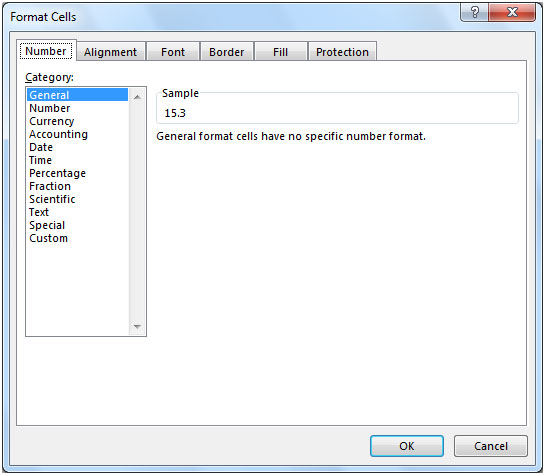• Select the Number tab, and from Category, select Number.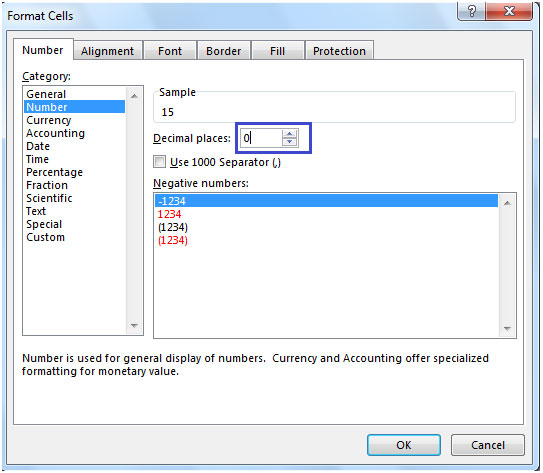• In Decimal places enter 0.

This is how the numbers look after formatting, see the below screenshot: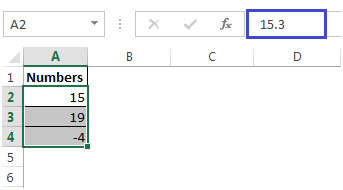We can also use the Round, Roundup & Rounddown functions to achieve this.

Let us take an example of each function to understand them.

ROUND: Rounds a number to a specified number of digits

Syntax =ROUND(number,num_digits)

Example of Round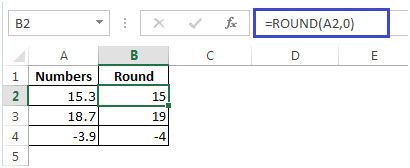In the above example A2 contains 15.3, formula in B2 will give us 15.

If the digits of the number you are rounding contain 5,6,7,8,9 then round the number up
e.g. 18.7 rounded to the nearest ten i.e. 19

If the digits of the number you are rounding contain 0,1,2,3,4 then round the number down.e.g. 15.3 rounded to the nearest ten i.e. 15

ROUNDUP: Rounds a number up, away from zero.

Syntax =ROUNDUP(number,num_digits)

Example of RoundUp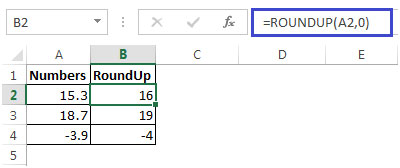ROUNDDOWN: Rounds a number down, toward zero

Syntax =ROUNDDOWN(number,num_digits)

Example of RoundDown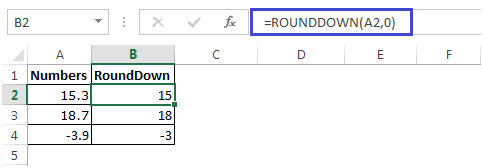1.Hello Experts,
I need a help of rounding of number. my requirement is as below.
Up to 50--> round to nearest to 1
50 to 100-->round to nearest to 5
greater than 100-->round to nearest 10

2.For rounding off to next 100 use (.. , -2)

3.Any way to round non standard in Excel. Example to round 430 to 400 and 431 to 500 ??

•Hey Steve,

Use the ROUNDDOWN function to round off 430 to 400. Formula =ROUNDDOWN (430,-2)
Use the ROUNDUP function to round off 431 to 500. Formula =ROUNDUP (430,-2)

•Cell A1 = number you want to round non-standard as above. Cell B1 = ROUND(A1+19,-2)

4.A better way would be to use the MROUND formula.

=MROUND(A2,50) would round up or down depending on which is closer.

•Thanks dear u solved my problem by using
CEILING : round the Number specified above up to the nearest multiple.

•Only if you want to round up or down at the same time. Those functions are good if there is an absolute cycle, i.e. a cycle that is of a specific time. So if the cycle starts, it runs (for example) 30 minutes, even if the value representing the cycle is between 0 and 30 minutes. For example 1.2 cycles is always 2 cycles; never rounded down to 1 cycle. The use of CEILING(1.2, 0.5) would make it work.

Terms and Conditions of use

The applications/code on this site are distributed as is and without warranties or liability. In no event shall the owner of the copyrights, or the authors of the applications/code be liable for any loss of profit, any problems or any damage resulting from the use or evaluation of the applications/code.